InfoQ 编辑部出品——2021年度技术盘点与展望 了解详情

为什么机器学习模型会失败？

• 2021 年 11 月 24 日
• 本文字数：4791 字

阅读完需：约 16 分钟模型训练

import pandas as pd

Unnamed: 0 kjkrfgld bpowgknt raksnhjf vwpsxrgk  omtioxzz  yfmzwkruid                                                                          29252  2225    KfoTG    zPfZR    DtMvg      NaN      12.0      -3.0   98286  1598    ljBjd    THHLT    DtMvg    esAQH      21.0      -2.0   49040  7896    Lsuai    zPfZR    zeYAm    ZCIYy      12.0      -3.0   35261  1458    KfoTG    mDadf    zeYAm    ZCIYy      12.0      -1.0   98833  1817    KfoTG    THHLT    DtMvg    ARuYG      21.0      -4.0
tiwrsloh weioazcf poor id 29252 -1.0 0.5 False 98286 -5.0 -9.5 True 49040 -5.0 -9.5 True 35261 -5.0 -9.5 False 98833 -5.0 -9.5 True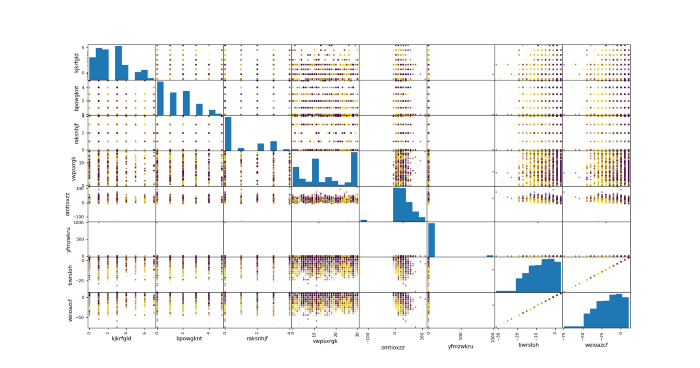支持向量机

from sklearn.pipeline import Pipelinefrom sklearn.preprocessing import RobustScalerfrom sklearn.neighbors import KNeighborsClassifier
model = Pipeline(steps=preprocess+[ ('scaler', RobustScaler()), ('estimator', KNeighborsClassifier(n_neighbors=5))])
model.fit(X_train, y_train)y_pred = model.predict(X_test)print(classification_report(y_test,y_pred))`

precision    recall  f1-score   support
False 0.73 0.77 0.75 891 True 0.70 0.66 0.68 750
accuracy 0.72 1641 macro avg 0.72 0.71 0.71 1641weighted avg 0.72 0.72 0.72 1641

KNN

from sklearn.pipeline import Pipelinefrom sklearn.preprocessing import RobustScalerfrom sklearn.neighbors import KNeighborsClassifier
model = Pipeline(steps=preprocess+[ ('scaler', RobustScaler()), ('estimator', KNeighborsClassifier(n_neighbors=5))])
model.fit(X_train, y_train)y_pred = model.predict(X_test)print(classification_report(y_test,y_pred))

precision    recall  f1-score   support
False 0.71 0.74 0.72 891 True 0.67 0.63 0.65 750
accuracy 0.69 1641 macro avg 0.69 0.69 0.69 1641weighted avg 0.69 0.69 0.69 1641

梯度提升

from sklearn.pipeline import Pipelinefrom sklearn.ensemble import GradientBoostingClassifier
model = Pipeline(steps=preprocess+[ ('estimator', GradientBoostingClassifier(max_depth=5, n_estimators=100))])
model.fit(X_train, y_train)y_pred = model.predict(X_test)print(classification_report(y_test,y_pred))

precision    recall  f1-score   support
False 0.76 0.78 0.77 891 True 0.73 0.70 0.72 750
accuracy 0.74 1641 macro avg 0.74 0.74 0.74 1641weighted avg 0.74 0.74 0.74 1641

模型预测分布

import matplotlib.pyplot as plt
pd.DataFrame(model.predict_proba(X_train)).hist()plt.show()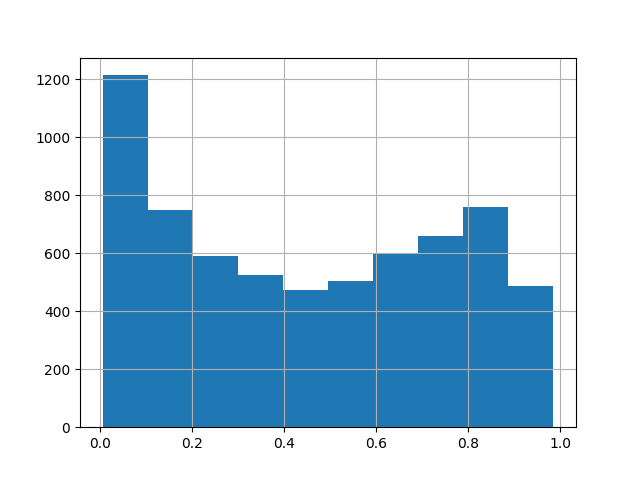pd.DataFrame(model.predict_proba(X_test)).hist()plt.show()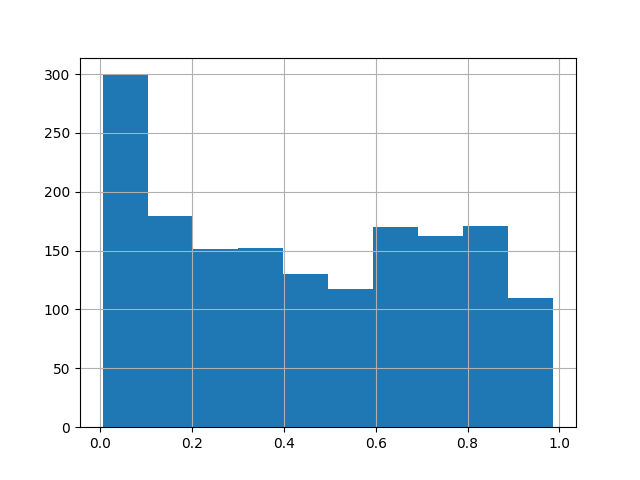偏差分布

train_proba = model.predict_proba(X_train)[:,1]pd.DataFrame(train_proba-y_train.astype(int)).hist(bins=50)plt.show()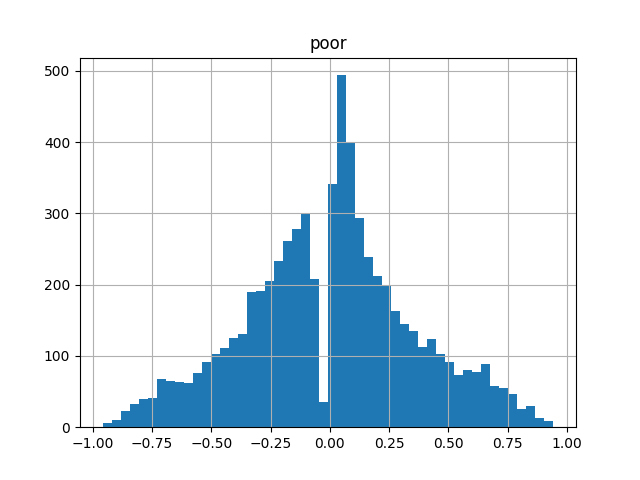test_proba = model.predict_proba(X_test)[:,1]pd.DataFrame(test_proba-y_test.astype(int)).hist(bins=50)plt.show()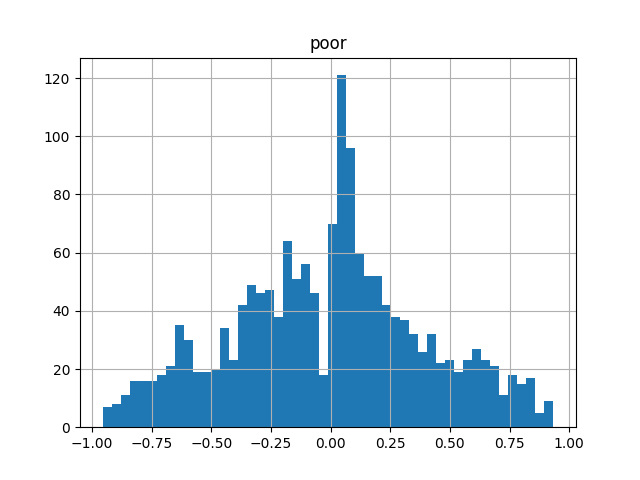验证正态性

import scipy
scipy.stats.normaltest(train_proba-y_train.astype(int))

NormaltestResult(statistic=15.602215177113427, pvalue=0.00040928141243470884)

模型可解释性

cols = X_train.columnsvals= dict(model.steps)['estimator'].feature_importances_
plt.figure()plt.bar(cols, vals)plt.show()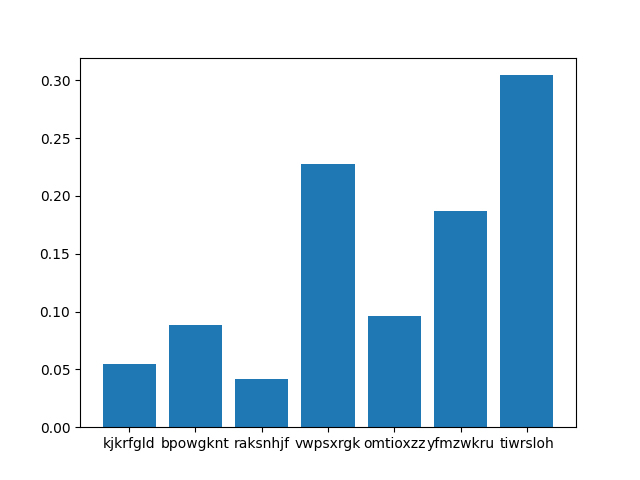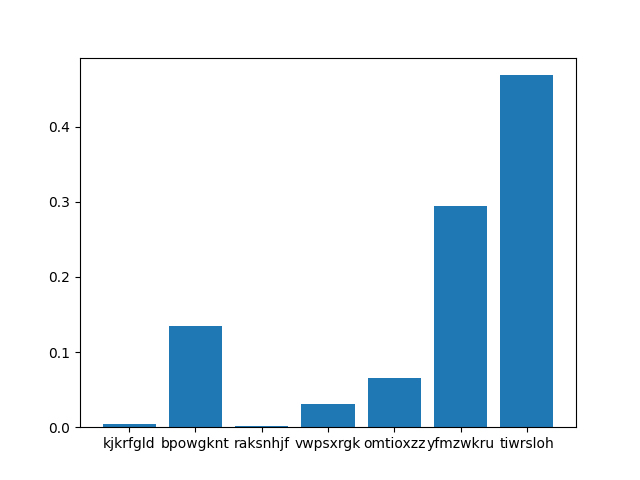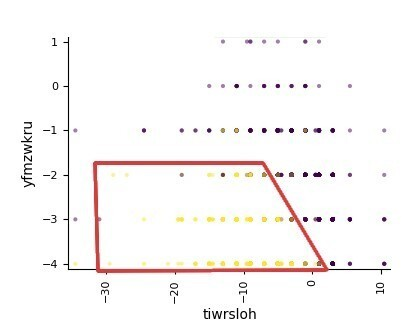我们学到了什么？

1. 尝试不同的函数族模型和超参数，并确认所有的下降在性能上处于平稳状态。

2. 为得到最好的解释，计算模型的输出分布和目标的偏差分布。如果数据是问题所在，则输出必须是均匀的，并且偏差必须遵循高斯分布。

3. 尽管数据是问题所在，但试着从模型输出和偏差分布中找到一个模型表现良好的区域。尝试对这个区域进行分割和定性，例如，用这个子集重新训练模型，并提取其可解释性。

4. 此外，在表征某些子集时，我们可以尝试从业务知识中思考，问题是来自数据的统计/测量噪音，还是来自缺乏预测因变量所需的一些特征值。

2021 年 11 月 24 日 10:00745评论

• AI 驱动的编码工具“CodeT5”来了：一种可以实时理解和生成代码的机器学习模型

AI驱动的编码工具，使用机器学习算法来根据输入数据生成代码，吸引了越来越多的关注。

• 如何使用 Python 进行超参调参和调优

超参数是所有机器学习和深度学习算法都包含的一部分。与由算法本身学习的标准机器学习参数（如线性回归中的w和b，或神经网络中的连接权重）不同，超参数由工程师在训练流程之前设置。

• 为什么大规模 Scrum 框架大都只是跟风，迟早会被放弃？

尽管我已经熟悉了许多大规模框架，但没有一次实践经验是正面的。

• 面向 CPU、GPU 和 IPU，英特尔发布重大技术架构的改变和创新

英特尔在Architecture Day 2021上发布了首个性能混合架构处理器Alder Lake，将有利于Windows 11的性能表现。

• DeepMind 新研究登上 Nature 封面，这一数学难题被 AI 攻破了

DeepMind 正在钻研纯数学。

• 如何从 0 到 1 搭建性能检测系统

不同的团队有着各自不同的业务，业务之间千差万别，性能指标也不能一概而论，所以用一套统一的检测模型覆盖所有场景是不现实的。本文将介绍如何定制一个属于自己团队的性能检测平台。

本周，又有大事儿了

• 新开发者入职的终极指南：行业最佳实践以及如何规划前 90 天的工作

如何让新开发者员工快速融入新的技术团队和工作环境？

12月日更

「架构实战营」

28天写作

「架构实战营」

电商系统微服务拆分

#架构实战营